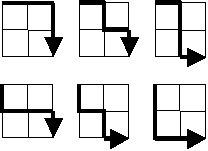# Counting Routes!

Probability Level 3The image shows the $6$ ways(routes) through which one can go from one point to the other in a $2 \times 2$ grid.

How many different routes can you find through a $4 \times4$ grid?

Note :This can be generalized for $n \times n$ grid

Also try

×